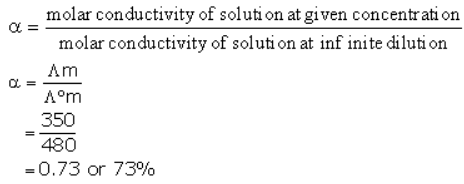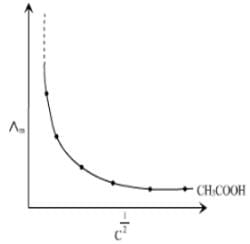# Test: Kohlrausch’s Law

## 10 Questions MCQ Test Chemistry Class 12 | Test: Kohlrausch’s Law

Description
Attempt Test: Kohlrausch’s Law | 10 questions in 15 minutes | Mock test for NEET preparation | Free important questions MCQ to study Chemistry Class 12 for NEET Exam | Download free PDF with solutions
QUESTION: 1

### Conductivity of a solution depends upon:

Solution:

The conductance of a solution depends upon its dilution, no. of ions present in solution. nature of solvent and nature of solute.
For example, with increases in dilution, the number of ions present in the solution increases and the conductance of the solution increases.

QUESTION: 2

### Limiting Ionic conductivity is defined as:

Solution:

The correct answer is option A

The molar conductivity of a solution at infinite dilution is known as limiting molar conductivity. In other words, When the concentration of the electrolyte approaches zero, the molar conductivity is known as limiting molar conductivity.

QUESTION: 3

### Which of the following statements is correct?

Solution:

The correct answer is Option D.

The molar conductivity increases with decrease in concentration both, for weak and strong electrolytes.

This is because the total volume, V, of a solution containing one mole of electrolyte also increases. It has been found that a decrease in k on dilution of a solution is more than compensated by an increase in its volume.

QUESTION: 4

Molar conductivity for a compound AB is 145.0 Scm2mol-1 and for CB is 110.1 Scm2mol-1. Limiting molar conductivity for A+ is 73.5 Scm2mol-1. What is limiting molar conductivity for C+?

Solution:

The correct answer is Option B.

Λm of AB = 145.05 cm2mol-1
Λm of AB = ΛoA + ΛoB (Kohlraush’s law)
145    = ΛoA + ΛoB --- (1)

Λm of CB = 110.10 Scm2mol-1

Λm of CB = ΛoC + Λo
110.1    =  ΛoC + ΛoB --- (2)

Eqn (1) - Eqn (2)

145 - 110.1 = ΛoA + ΛoB - ΛoC + ΛoB
34.9 = ΛoA - ΛoC
34.9 = 73.5 - ΛoC
ΛoC = 73.5 - 34.9
= 38.6 Scm2mol-1

QUESTION: 5

Kohlrausch’s Law shows that:

Solution:

The correct answer is option A

Kohlrausch's law states that the equivalent conductivity of an electrolyte at infinite dilution is equal to the sum of the conductances of the anions and cations. If a salt is dissolved in water, the conductivity of the solution is the sum of the conductances of the anions and cations.
Hence, at infinite dilution the ionic conductivity of ions is additive.

QUESTION: 6

Conductivity of a solution contained within 1 centimetre cube volume is known as:

Solution:

The correct answer is option C
Conductivity of a solution is equal to the conductance of a solution of 1cm length and cross section area of 1 square cm it may also be defined as the conductance of one centimeter cube of the conductor .It is represented by the symbol kappa (k) mathematically we can write
K = G×G⋅ conductance ,G⋅=cell constant
The unit of K is ohm−1cm−1 or S cm−1
The conductivity K of an electrolytic solution depends on the concentration of the electrolyte nature of solvent and temperature .molar conductivity molar conductivity of a solution at a given concentration is the conductance of the volume v of solution containing one mole of electrolyte between two electrodes with area of cross section a and distance of unit length.

QUESTION: 7

Kohlrausch’s Law is applicable:

Solution:

The correct answer is Option D.

Kohlrausch's law states that the equivalent conductivity of an electrolyte at infinite dilution is equal to the sum of the conductances of the anions and cations. When the concentration of the electrolyte approaches zero, the molar conductivity is known as limiting molar conductivity, Ëm°

QUESTION: 8

At 300K molar conductivity of solution A is 350 units, and at infinite dilution the molar conductivity of the same sample is 480 unit. Predict the percentage dissociation of the electrolyte.

Solution:QUESTION: 9

For weak electrolytes ∧mo cannot to be calculated by extrapolation of ∧mo vs (c)1/2graph. Which of the following is not the reason?

Solution:

The correct answer is option  B
In case of the weak electrolyte it is not possible to calculate the molar conductivity at infinite dilution by extrapolation of the graph between the molar conductivity and the concentration,because there is large variation between them. As you can see from the graph:-In case of weak electrolytes conductivity can be calculated by Kohlrausch law.

QUESTION: 10

With increase in temperature the degree of dissociation of electrolyte:

Solution:Use Code STAYHOME200 and get INR 200 additional OFF Use Coupon Code